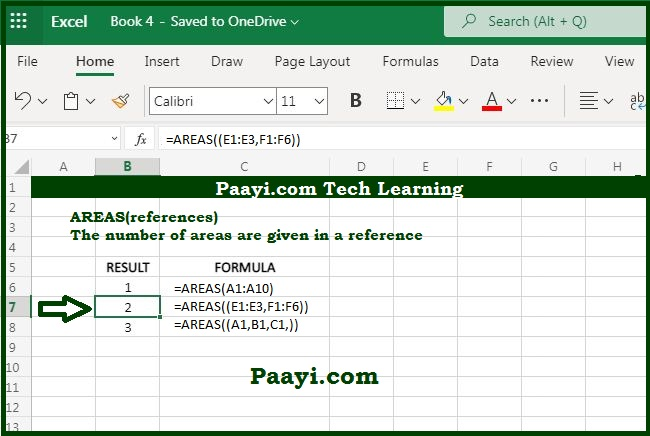# Learn How to Use Microsoft Excel AREAS Function

Written by | 0 Comments | 668 Views

In this article, you will learn how to use the Microsoft Excel AREAS function and its prime function in Microsoft Excel. You will also get to know the Microsoft Excel AREAS function return value and syntax with the help of some examples.

## Microsoft Excel AREAS Function

The main objective of the Microsoft Excel AREAS function is to get the number of areas in a reference. So, you able to return the number of areas in a provided reference with the help of the AREAS function. So, when we use the formula = AREAS((A1:C1, A2:C2)), the return value will be 2. In case you want to use multiple references, they should be enclosed in the extra set of parenthesis.

### AREAS Function Return Value

The return value will be the number representing a number of areas.

### AREAS Syntax

= AREAS(reference)

Where the argument:

• reference: It is the reference of a particular cell or the range of cells.

## How to Use Microsoft Excel AREAS Functions?You can include more than one reference in the AREAS function and separate the multiple references using a comma and enclose them in an extra parenthesis set. Otherwise, Excel will regard commas as multiple arguments and generate an error.